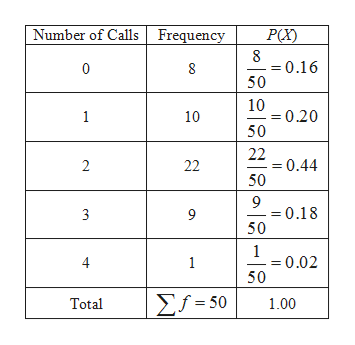# The information below is the number of daily emergency service calls made by the volunteer ambulance service of Walterboro, South Carolina, for the last 50 days. To explain, there were 22 days on which there were two emergency calls, and 9 days on which there were three emergency calls. Number of CallsFrequency081102223941Total50 a. Convert this information on the number of calls to a probability distribution. (Round your answers to 2 decimal places.)Number of calls               Probability01234b. Is this an example of a discrete or continuous probability distribution?

Question
171 views

The information below is the number of daily emergency service calls made by the volunteer ambulance service of Walterboro, South Carolina, for the last 50 days. To explain, there were 22 days on which there were two emergency calls, and 9 days on which there were three emergency calls.

 Number of Calls Frequency 0 8 1 10 2 22 3 9 4 1 Total 50

a. Convert this information on the number of calls to a probability distribution. (Round your answers to 2 decimal places.)

Number of calls               Probability

0

1

2

3

4

b. Is this an example of a discrete or continuous probability distribution?

check_circle

Step 1

(a)

The number of calls to a probability distribution is obtained below:

The required formula for the probability distribution for the random variable x is,

Step 2

The probability distribution for the random v...help_outlineImage TranscriptioncloseNumber of Calls Frequency P(X) 8 0.16 50 0 10 0.20 50 1 10 22 0.44 50 2 22 9 = 0.18 50 3 9 1 = 0.02 50 1 Σ1-50 Total 1.00 fullscreen

### Want to see the full answer?

See Solution

#### Want to see this answer and more?

Solutions are written by subject experts who are available 24/7. Questions are typically answered within 1 hour.*

See Solution
*Response times may vary by subject and question.
Tagged in

### Other